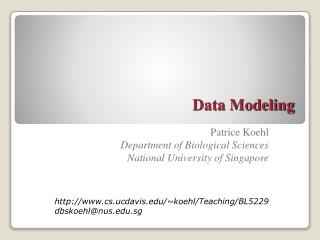DownloadDownload PresentationData Modeling

# Data Modeling

Download Presentation## Data Modeling

- - - - - - - - - - - - - - - - - - - - - - - - - - - E N D - - - - - - - - - - - - - - - - - - - - - - - - - - -
##### Presentation Transcript

1. Data Modeling Patrice Koehl Department of Biological Sciences National University of Singapore http://www.cs.ucdavis.edu/~koehl/Teaching/BL5229 dbskoehl@nus.edu.sg

2. Data Modeling • Data Modeling: least squares • Data Modeling: Non linear least squares • Data Modeling: robust estimation

3. Data Modeling • Data Modeling: least squares • Linear least squares • Data Modeling: Non linear least squares • Data Modeling: robust estimation

4. Least squares Suppose that we are fitting N data points (xi,yi) (with errors si on each data point) to a model Y defined with M parameters aj: The standard procedure is least squares: the fitted values for the parameters aj are those that minimize: Where does this come from?

5. Least squares • Let us suppose that: • The data points are independent of each other • Each data point as a measurement error that is random, distributed as a • Gaussian distribution around the “true” value Y(xi) • The probability of the data points, given the model Y is then:

6. Least squares Application of Bayes ‘s theorem: With no information on the models, we can assume that the prior probability P(Model) is constant. Finding the coefficients a1,…aM that maximizes P(Model/Data) is then equivalent to finding the coefficients that maximizes P(Data/Model). This is equivalent to maximizing its logarithm, or minimizing the negative of its logarithm, namely:

7. Fitting data to a straight line

8. Fitting data to a straight line This is the simplest case: Then: The parameters a and b are obtained from the two equations:

9. Fitting data to a straight line Let us define: then a and b are given by:

10. Fitting data to a straight line We are not done! Uncertainty on the values of a and b: Evaluate goodness of fit: • Compute c2 and compare to N-M (here N-2) • Compute residual error on each data point: Y(xi)-yi • Compute correlation coefficient R2

11. Fitting data to a straight line

12. General Least Squares Then: The minimization of c2 occurs when the derivatives of c2 with respect to the parameters a1,…aMare 0. This leads to M equations:

13. General Least Squares Define design matrix A such that

14. General Least Squares Define two vectors b and a such that and a contains the parameters Note that c2 can be rewritten as: The parameters a that minimize c2 satisfy: These are the normal equations for the linear least square problem.

15. General Least Squares How to solve a general least square problem: 1) Build the design matrix A and the vector b 2) Find parameters a1,…aMthat minimize (usually solve the normal equations) 3) Compute uncertainty on each parameter aj: if C = ATA, then

16. Data Modeling • Data Modeling: least squares • Data Modeling: Non linear least squares • Data Modeling: robust estimation

17. Non linear least squares In the general case, g(X1,…,Xn) is a non linear function of the parameters X1,…Xn; c2 is then also a non linear function of these parameters: Finding the parameters X1,…,Xn is then treated as finding X1,…,Xn that minimize c2.

18. Minimizing c2 Some definitions: Gradient: The gradient of a smooth function f with continuous first and second derivatives is defined as: Hessian The n x n symmetric matrix of second derivatives, H(x), is called the Hessian:

19. Minimizing c2 Minimization of a multi-variable function is usually an iterative process, in which updates of the state variable x are computed using the gradient and in some (favorable) cases the Hessian. Steepest descent (SD): The simplest iteration scheme consists of following the “steepest descent” direction: Usually, SD methods leads to improvement quickly, but then exhibit slow progress toward a solution. They are commonly recommended for initial minimization iterations, when the starting function and gradient-norm values are very large. (a sets the minimum along the line defined by the gradient)

20. Minimizing c2 Conjugate gradients (CG): In each step of conjugate gradient methods, a search vector pk is defined by a recursive formula: The corresponding new position is found by line minimization along pk: the CG methods differ in their definition of .

21. Minimizing c2 Newton’s methods: Newton’s method is a popular iterative method for finding the 0 of a one-dimensional function: x3 x2 x1 x0 It can be adapted to the minimization of a one –dimensional function, in which case the iteration formula is:

22. Minimizing c2 The equivalent iterative scheme for multivariate functions is based on: Several implementations of Newton’s method exist, that avoid Computing the full Hessian matrix: quasi-Newton, truncated Newton, “adopted-basis Newton-Raphson” (ABNR),…

23. Data analysis and Data Modeling • Data Modeling: least squares • Data Modeling: Non linear least squares • Data Modeling: robust estimation

24. Robust estimation of parameters Least squares modeling assume a Gaussian statistics for the experimental data points; this may not always be true however. There are other possible distributions that may lead to better models in some cases. One of the most popular alternatives is to use a distribution of the form: Let us look again at the simple case of fitting a straight line in a set of data points (ti,Yi), which is now written as finding a and b that minimize: b = median(Y-at) and a is found by non linear minimization

25. Robust estimation of parameters